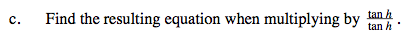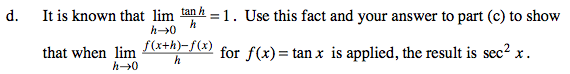### Home > PC > Chapter 9 > Lesson 9.2.2 > Problem9-86

9-86.
1. Challenge: Given f(x) = tan x. Homework Help ✎

1. Use the fact that sin(x +y) = sin x cos y + cos x sin y
and cos(x + y) = cos x cos y − sin x sin y to show that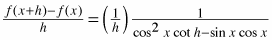.

2. At this point, can you find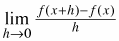? Explain.

3. Find the resulting equation when multiplying by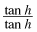.

4. It is known that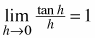. Use this fact and your answer to part (c) to show that whenfor f(x) = tan x is applied, the result is sec2x.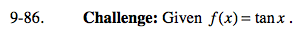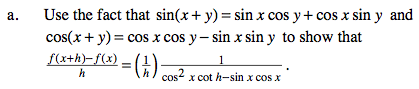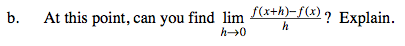No, you would have division by 0.